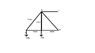# Finding the unknown forces at two supporting pins

• ptguard1

## Homework Statement

In a simple truss where P = 8 kip, find the unknown forces D_x, D_y, C_x, and C_y then determine the average normal stress in each member.

Description of truss:

I cannot copy an image due to the source it is coming from, so here is my attempt at a description

A triangle with arms AB (going up and to the right (5 feet)), BD (going down and to the right(5 feet)), and DA (going to the left connecting back with A (8 feet)). Member BE cutting straight down the middle of the triangle (3 feet). Member BC parallel with member ED and equal length of 4 feet. Pins at C and D. The force P is applied downward at point A and the force 0.75P is applied downward at point E.

## The Attempt at a Solution

I know that in a 2D problem you can only have three unknowns to find the forces. I am able to find C_x and D_x to be 29.3 kip (D_x in the negative x direction), but cannot remember how to find the y values.

#### Attachments

•Truss.png
4.3 KB · Views: 484
Last edited: